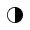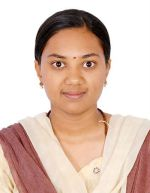• Resize textA -AA +
•Home  »  PMR Fellowship Report 2

# WRITE-UP FOR PMR FELLOWSHIP FROM FEBRUARY 2021 - OCTOBER 2021## Harshitha C

Our main focus during the past one year was to learn foundational material related to the theory of representations of p-adic groups, the subject area of our interest. As a part of this, we undertook several reading projects.

1.  Representations of finite groups: Basics of representation theory of finite groups was covered. We also studied the construction of all complex irreducible representations of the group GL2(F) where F is a finite field.

2. Representations of compact groups: We studied the theory of representations of compact groups. Our main aim was to understand the proof of Peter-Weyl theorem which showed that the space of matrix coefficients is dense in the space of continuous functions on a compact group.

3. Basic algebraic number theory: We studied the basic results of classical algebraic number theory. After getting a basic understanding of the structure of ring of integers of an algebraic number field, we studied some famous fundamental theorems in the subject like unique factorization of ideals in the ring of integers, finiteness of class number and Dirichlet’s unit theorem. We next turned our attention to the local theory. We studied the structure, classification and properties of locally compact non-discrete fields (local fields).

4. Tate’s thesis: As an introduction to the theory of automorphic forms, we read Tate’s thesis. We began with the study of dual of locally compact groups and their properties. This was applied to understand in particular the dual of local fields and the adele group of global fields. The isomorphism obtained on the Schwartz-Bruhat spaces via the Fourier transform was used to prove the analytic continuation and functional equation of the local and global zeta functions as defined by Tate in his thesis. This was used to give an alternate proof of the functional equation and analytic continuation of classical zeta functions considered by Hecke. As a consquence, analytic continuation and functional equations of some well known L-functions like Riemann zeta function, Dedekind zeta function etc were obtained.

5. Representatons of p-adic groups: We started studying the complex representations of l-groups, which are locally compact Hausdorff groups which have a fundamental system of neighborhoods of the identity consisting of compact and open subgroups. After looking into the generalities in the subject like the notion of smooth and admissible representations and their basic properties, we studied the properties of two fundamental functors namely smooth induction and Jacquet functor. We also studied the proof that every smooth irreducible representation of general linear group over a local field is admissible which uses the above mentioned properties.

Future plan:

We plan to continue studying the theory of representations of p-adic groups, in particular the representation theory of the general linear group over a non-archimedean local field F. In particular, we would like to understand the Zelevinksy and Langlands classification of irreducible admissible representations of GLn(F).

Courses attended:

MTH 611- Algebra-1

MTH 612- Analysis-1

MTH 613- Topology-1

MTH 621- Algebra-2

MTH 623- Topology-2

MTH 627- Differential Geometry

Teaching assistantship:

MTH 211- Multivariable Calculus

MTH 221- Probability and Statistics

MTH 314- Linear Algebra